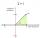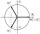# Complex number coordinates

Which coordinates show the location of -2+3i

Result

x =  -2
y =  3

#### Solution:Leave us a comment of example and its solution (i.e. if it is still somewhat unclear...):

Showing 0 comments:Be the first to comment!#### To solve this example are needed these knowledge from mathematics:

Try our complex numbers calculator.

## Next similar examples:

1. Imaginary numbersFind two imaginary numbers whose sum is a real number. How are the two imaginary numbers related? What is its sum?
2. Im>0?Is -10i a positive number?
3. Linear imaginary equationGiven that ? "this is z star" Find the value of the complex number z.
4. ABS CNCalculate the absolute value of complex number -15-29i.
5. ReciprocalCalculate reciprocal of z=0.8-1.8i:
6. De Moivre's formulaThere are two distinct complex numbers z such that z3 is equal to 1 and z is not equal 1. Calculate the sum of these two numbers.
7. Is complexAre these numbers 2i, 4i, 2i + 1, 8i, 2i + 3, 4 + 7i, 8i, 8i + 4, 5i, 6i, 3i complex?
8. Theorem proveWe want to prove the sentense: If the natural number n is divisible by six, then n is divisible by three. From what assumption we started?
9. TrinityHow many different triads can be selected from the group 43 students?
10. Fish tankA fish tank at a pet store has 8 zebra fish. In how many different ways can George choose 2 zebra fish to buy?
11. Calculation of CNCalculate: ?
12. SequenceBetween numbers 1 and 53 insert n members of the arithmetic sequence that its sum is 702.
13. 75th percentile (quartille Q3)Find 75th percentile for 30,42,42,46,46,46,50,50,54
14. Geometric progression 2There is geometric sequence with a1=5.7 and quotient q=-2.5. Calculate a17.
15. EquationEquation ? has one root x1 = 8. Determine the coefficient b and the second root x2.
16. Linsys2Solve two equations with two unknowns: 400x+120y=147.2 350x+200y=144
17. DiscriminantDetermine the discriminant of the equation: ?